# NEET Previous Year Questions (2014-21): Dual Nature of Radiation & Matter Notes | Study Physics Class 12 - NEET

## NEET: NEET Previous Year Questions (2014-21): Dual Nature of Radiation & Matter Notes | Study Physics Class 12 - NEET

The document NEET Previous Year Questions (2014-21): Dual Nature of Radiation & Matter Notes | Study Physics Class 12 - NEET is a part of the NEET Course Physics Class 12.
All you need of NEET at this link: NEET

Q.1. The number of photons per second on an average emitted by the source of monochromatic light of wavelength 600 nm, when it delivers the power of 3.3 × 10–3 watt will be : (h = 6.6 × 10–34 Js)      (2021)
A: 1016
B: 1015
C: 1018
D: 1017
Ans:
A
Solution: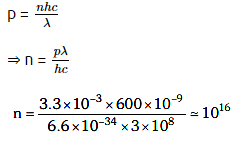Q.2. An electromagnetic wave of wavelength 'λ' is incident on a photosensitive surface of negligible work function. If 'm' is mass of photoelectron emitted from the surface has de-Broglie wavelength λd, then:      (2021)
A: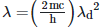B: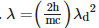C: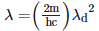D:Ans: A
Solution:Q.3. Light of frequency 1.5 times the threshold frequency is incident on a photosensitive material. What will be the photoelectric current if the frequency is halved and intensity is doubled?      (2020)
A: one-fourth
B: zero

C: doubled
D: four times

Ans: B
Solution:
f0 < f1 = 1.5 f0
∴ f2 = 0.75 f0
for given condition
fincident < fthreshold
so no photo electron emission
i = 0

Q.4. An electron is accelerated through a potential difference of 10,000 V. Its de-Broglie wavelength is, (nearly) : (me = 9 × 10–31 kg)     (2019)
A: 12.2 × 10–13 m
B: 12.2 × 10–12 m
C: 12.2 × 10–14 m
D: 12.2 nm
Ans: B
Solution:

For an electron accelerated through a potential V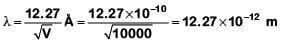Q.5. An electron of mass m with an initial velocity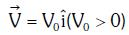enters an electric field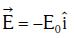E0 = constant > 0) at t = 0. If λ0 is its de-Broglie wavelength initially, then its de-Broglie wavelength at time t is :-    (2018)
A: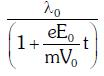B: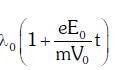C: λ0t
D: λ0
Ans:
A
Solution: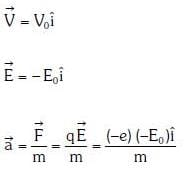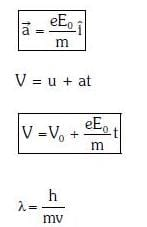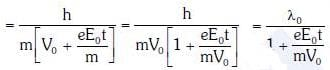Q.6. When the light of frequency 2v0 (where v0 is threshold frequency), is incident on a metal plate, the maximum velocity of electrons emitted is v1. When the frequency of the incident radiation is increased to 5 v0, the maximum velocity of electrons emitted from the same plate is v2. The ratio of v1 to v2 is :-     (2018)
A: 1 : 2
B: 1 : 4
C: 4 : 1
D: 2 : 1
Ans:
A
Solution: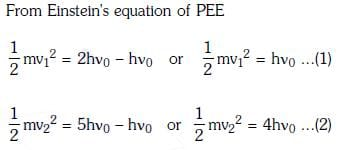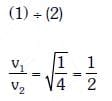Q.7. The photoelectric threshold wavelength of silver is 3250 × 10–10m. The velocity of the electron ejected from a silver surface by ultraviolet light of wavelength 2536 × 10–10 m is :-     (2017)
(Given h = 4.14 × 10–15 eVs and c = 3 × 108 ms–1)
A: ≈ 0.6 × 106 ms–1
B: ≈ 61 × 103 ms–1
C: ≈ 0.3 × 106 ms–1
D: ≈ 6 × 105 ms
–1
Ans: A
Solution: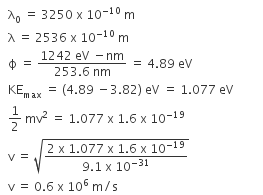Q.8. When a metallic surface is illuminated with radiation of wavelength λ the stopping potential is V. If the same surface is illuminated with radiation of wavelength 2λ, the stopping potential is V/4. The threshold wavelength for the metallic surface is :    (2016)
A: 3λ
B: 4λ
C: 5λ
D: 5/2λ
Ans:
A
Solution:
When a metallic surface is illuminated with radiation of wavelength λ, the stopping potential is V.
Photoelectric equation can be written as,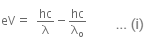Now, when the same surface is illuminated with radiation of wavelength 2λ, the stopping potential is V/4. So, photoelectric equation can be written as,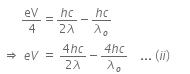From equations (i) and (ii), we get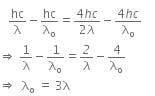When a metallic surface is illuminated with radiation of wavelength λ stopping potential is V.
Photoelectric equation can be written as,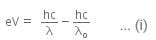Now, when the same surface is illuminated with radiation of wavelength 2λ, the stopping potential is V/4. So, photoelectric equation can be written as,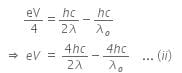From equations (i) and (ii), we get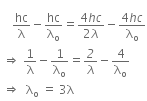Q.9. An electron of mass m and a photon have same energy E. The ratio of de-Broglie wavelengths associated with them is:    (2016)
A: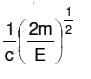B: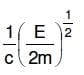C: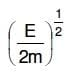D: c(2mE)1/2
Ans:
B
Solution:

Given that electron has a mass m.
De-Broglie wavelength for an electron will be given as,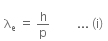where,
h is the Planck's constant, and
p is the linear momentum of electron
Kinetic energy of electron is given by,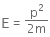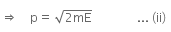From equation (i) and (ii), we have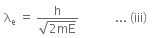Energy of a photon can be given as,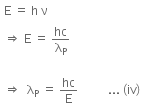Hence, λP is the de-Broglie wavelength of photon.
Now, dividing equation (iii) by (iv), we get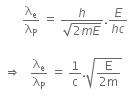Q.10. A certain metallic surface is illuminated with monochromatic light of wavelength, λ. The stopping potential for photo-electric current for this light is 3V0. If the same surface is illuminated with light of wavelength 2λ, the stopping potential is V0. The threshold wavelength for this surface for photoelectric effect is :    (2015)
A: λ/6
B: 6λ
C: 4λ
D: λ/4
Ans:
C
Solution:
We have,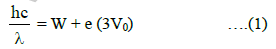where W is the work function and (3V0) is the stopping potential when monochromatic light of wavelength λ is used.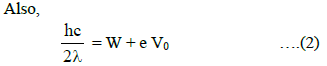where V0 is the stopping potential when monochromatic light of wavelength 2λ is used.
Subtracting equation (2) from equation (1)
We get,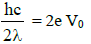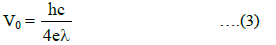Substituting in equation (2) we get,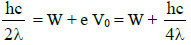∴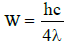The threshold wavelength is therefore 4λ.

Q.11. Which of the following figures represent the variation of particle momentum and the associated de-Broglie wavelength?    (2015)
A: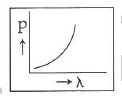B: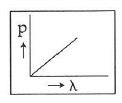C: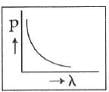D: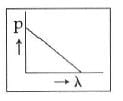Ans:
C
Solution:

The de-Broglie wavelength is given by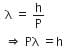This equation is in the form of yx = c, which is the equation of a rectangular hyperbola.
The de-Broglie wavelength is given by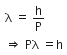This equation is in the form of yx = c, which is the equation of a rectangular hyperbola.

Q.12. When the energy of the incident radiation is increased by 20%, the kinetic energy of the photoelectrons emitted from a metal surface increased from 0.5 eV to 0.8 eV. The work function of the metal is :     (2014)
A: 1.3 eV
B: 1.5 eV
C: 0.65 eV
D: 1.0 eV
Ans:
D
Solution:
Original energy of photon be E0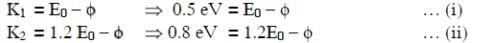From equation (i) and (ii)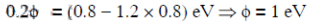Q.13. If the kinetic energy of the particle is increased to 16 times its previous value, the percentage change in the de−Broglie wavelength of the particle is :    (2014)
A: 60
B: 50
C: 25
D: 75
Ans:
D
Solution: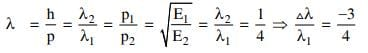The document NEET Previous Year Questions (2014-21): Dual Nature of Radiation & Matter Notes | Study Physics Class 12 - NEET is a part of the NEET Course Physics Class 12.
All you need of NEET at this link: NEETUse Code STAYHOME200 and get INR 200 additional OFF

## Physics Class 12

134 videos|388 docs|213 tests

### How to Prepare for NEET

Read our guide to prepare for NEET which is created by Toppers & the best Teachers

Track your progress, build streaks, highlight & save important lessons and more!

,

,

,

,

,

,

,

,

,

,

,

,

,

,

,

,

,

,

,

,

,

;